# R S Aggarwal Solutions for Class 11 Maths Chapter 31 Probability

R S Aggarwal Solutions Class 11 Chapter 31 are given here which consist of questions and answers related to Probability. Probability is a measure of the possibility that an event will occur. Practicing from R S Aggarwal solutions will help students to score good marks in exams.

Important terms and their definitions:

Experiment: An operation which can produce some well defined outcomes.

Random Experiment: If in each trial of an experiment, conducted under identical conditions, the outcome is not unique, but may be any of the several possible outcomes, then such an experiment is known as random experiment.

Sample Space: The set of all possible outcomes in a random experiment.

Event: Every subset of a sample space.

Compound event: An event which is not simple.

Mutually exclusive events: Two events A and B are said to be mutually exclusive if A n B = Φ.

## R S Aggarwal Solutions for Chapter 31 Probability

R S Aggarwal Chapter 31 – Probability, contains the answers to all the questions listed in this chapter. Let us have a look at some of the topics covered here:

• Probability Introduction
• Sample Space
• Events
• Mutually Exclusive events
• Probability of an event
• Theorems for two and three events

## Download PDF of R S Aggarwal Solutions for Class 11 Maths Chapter 31 Probability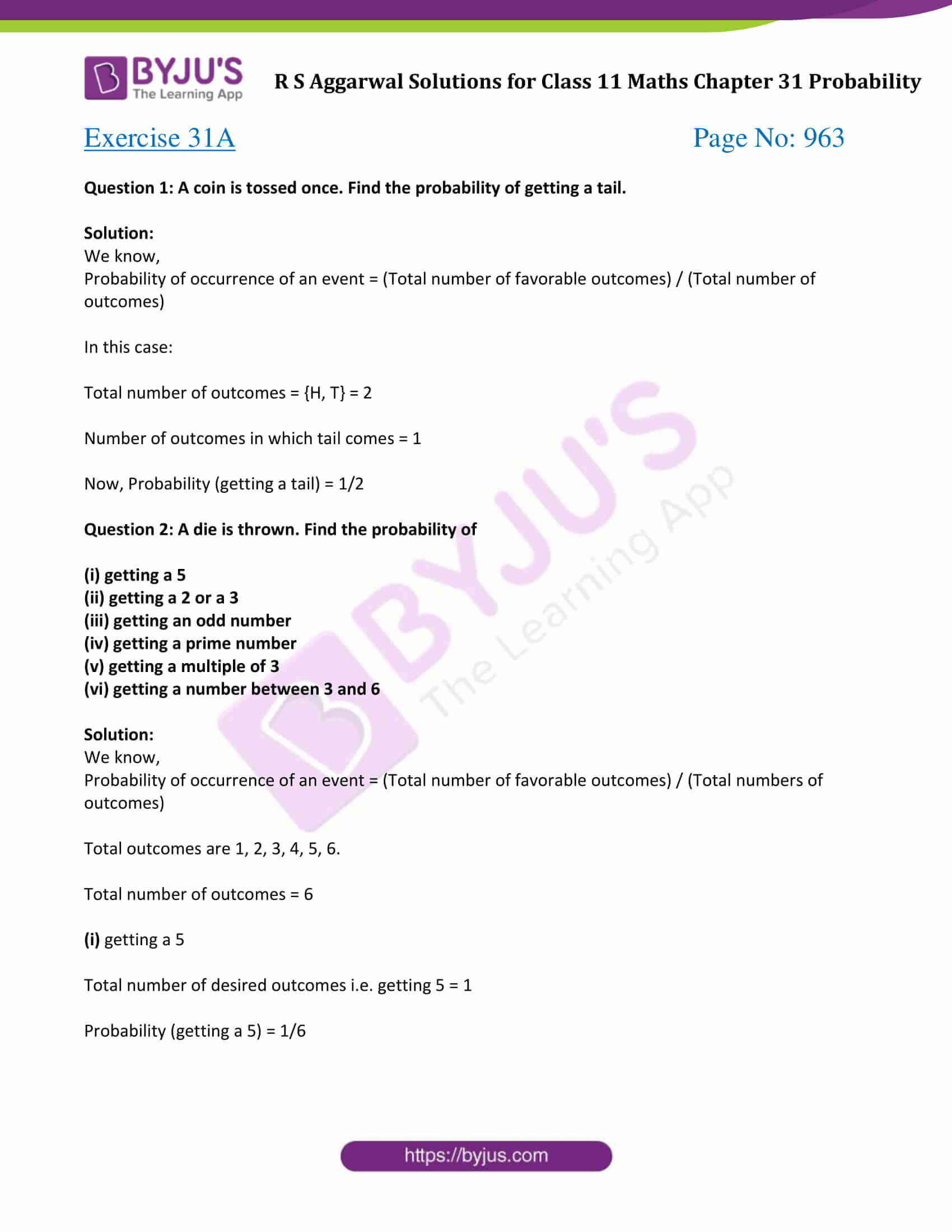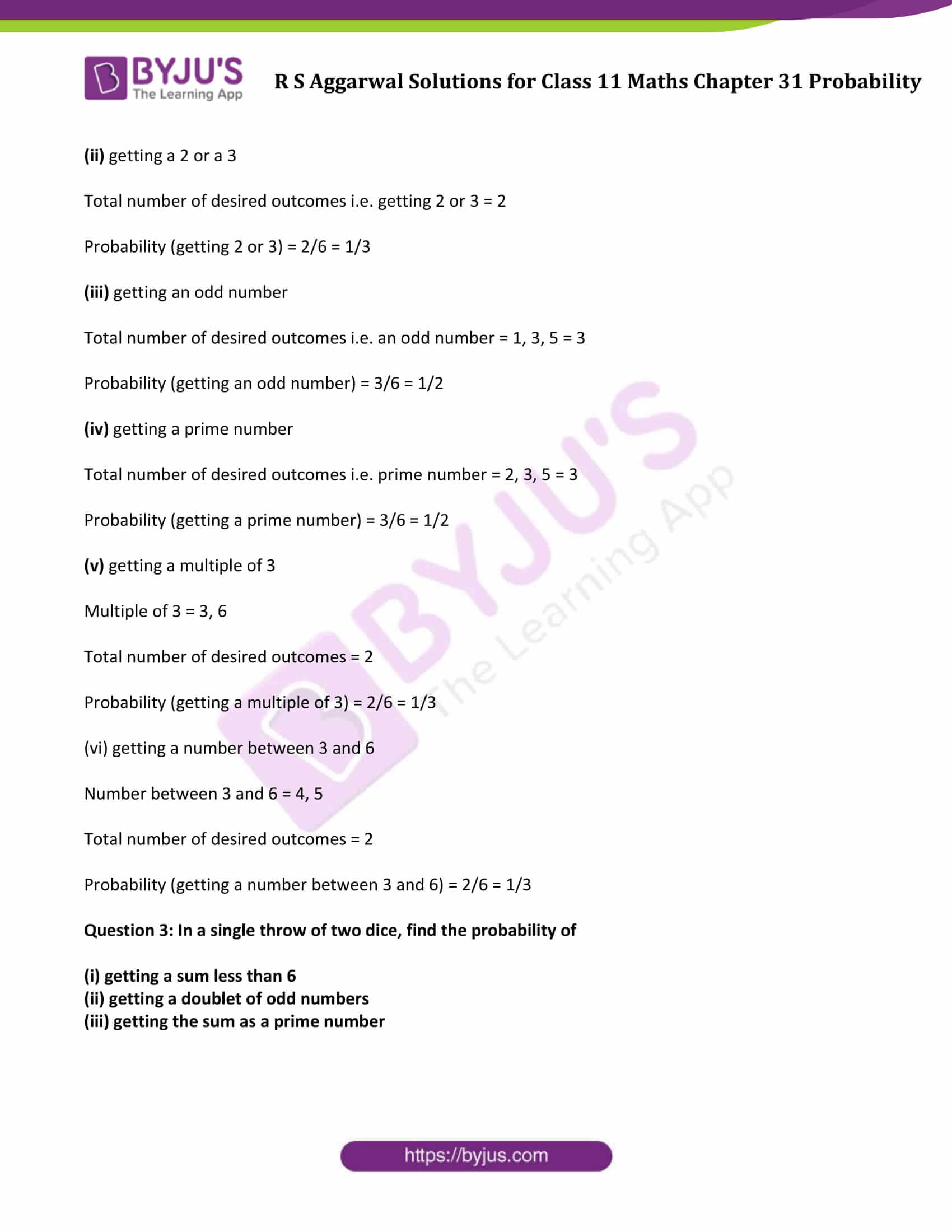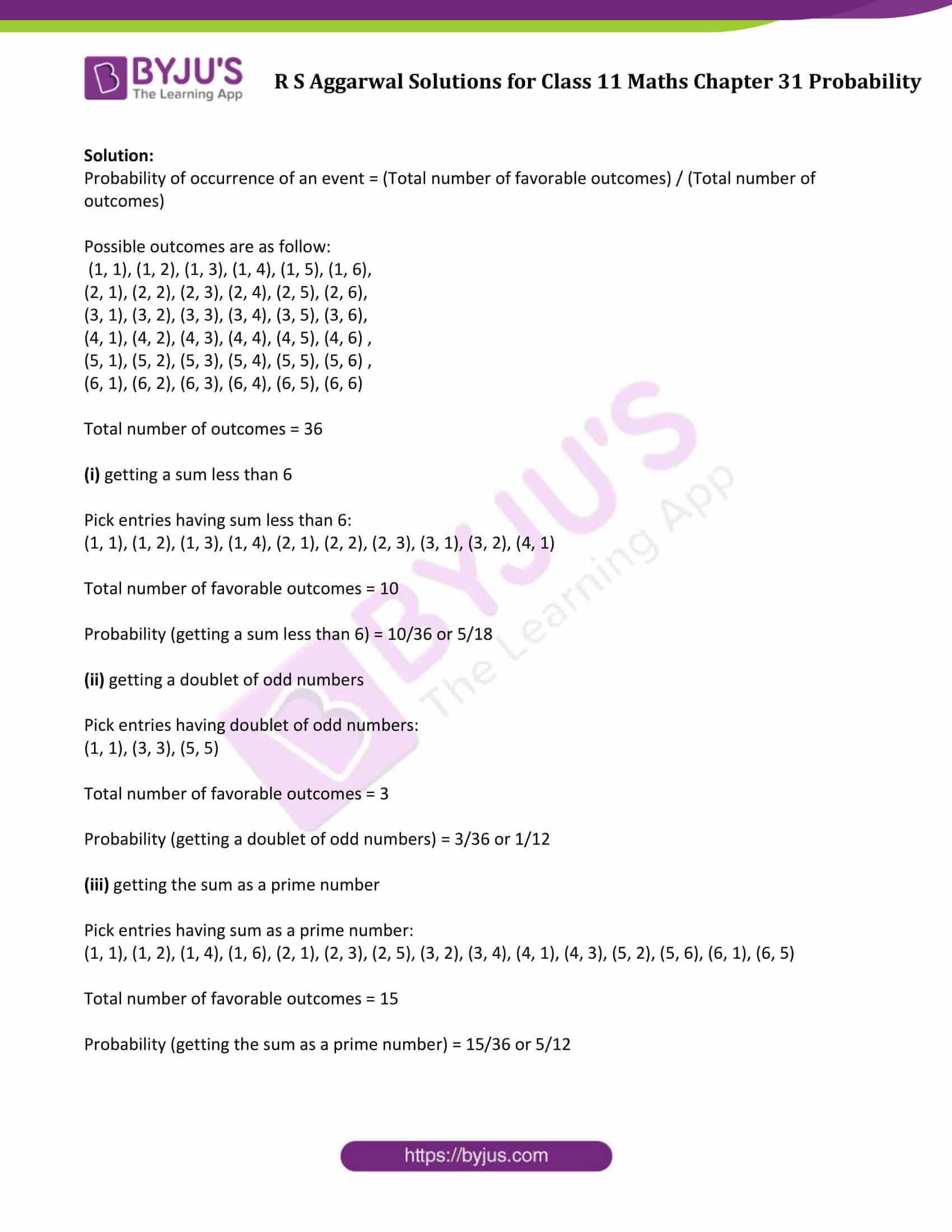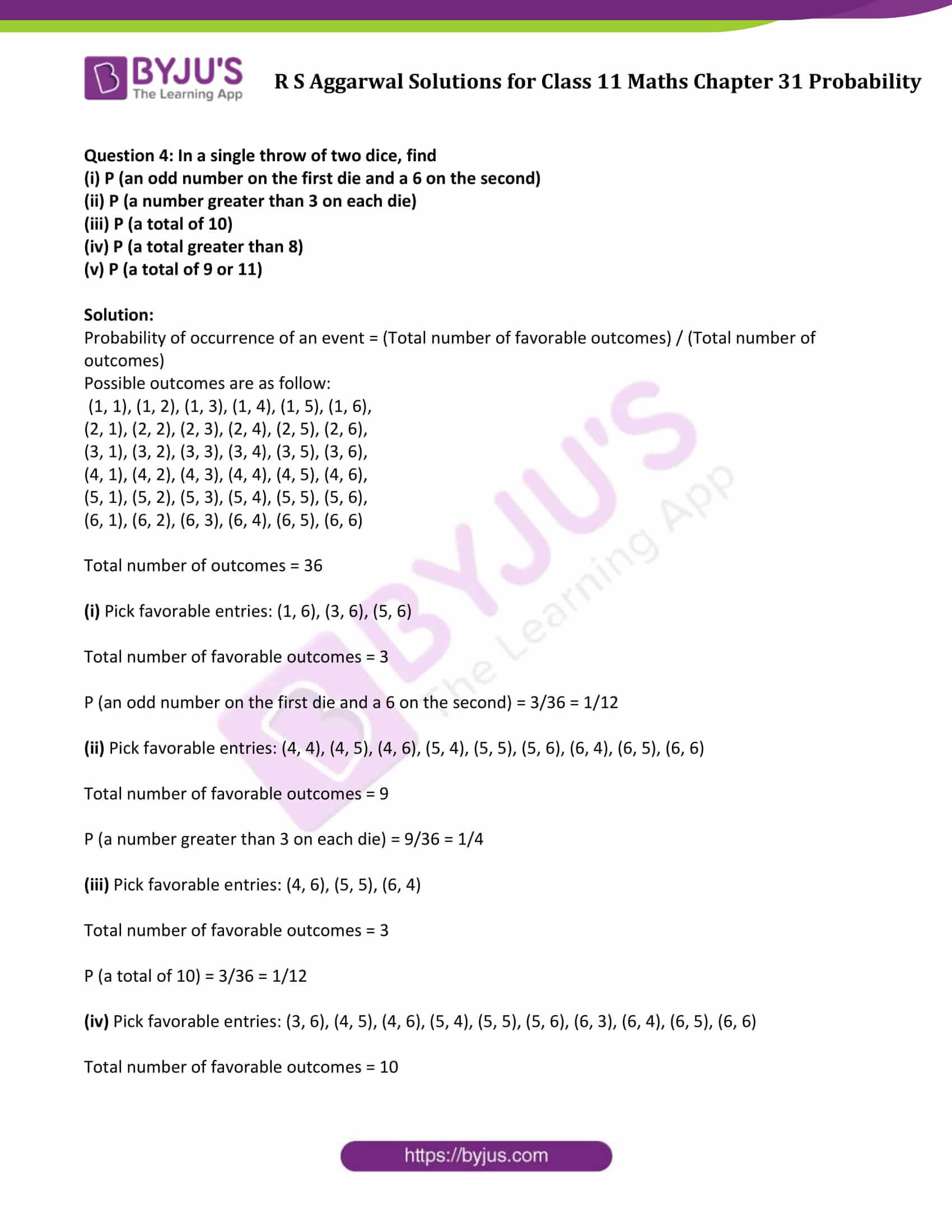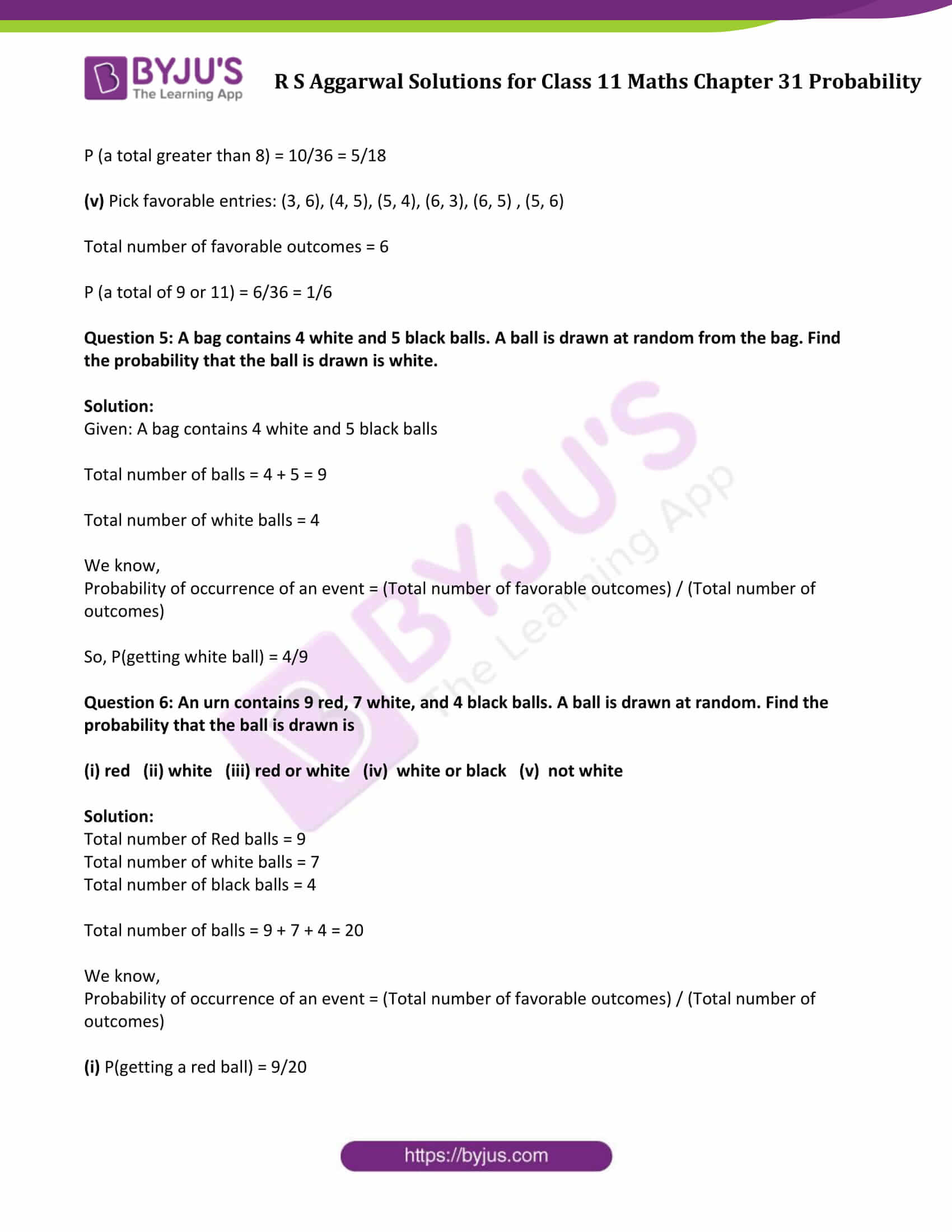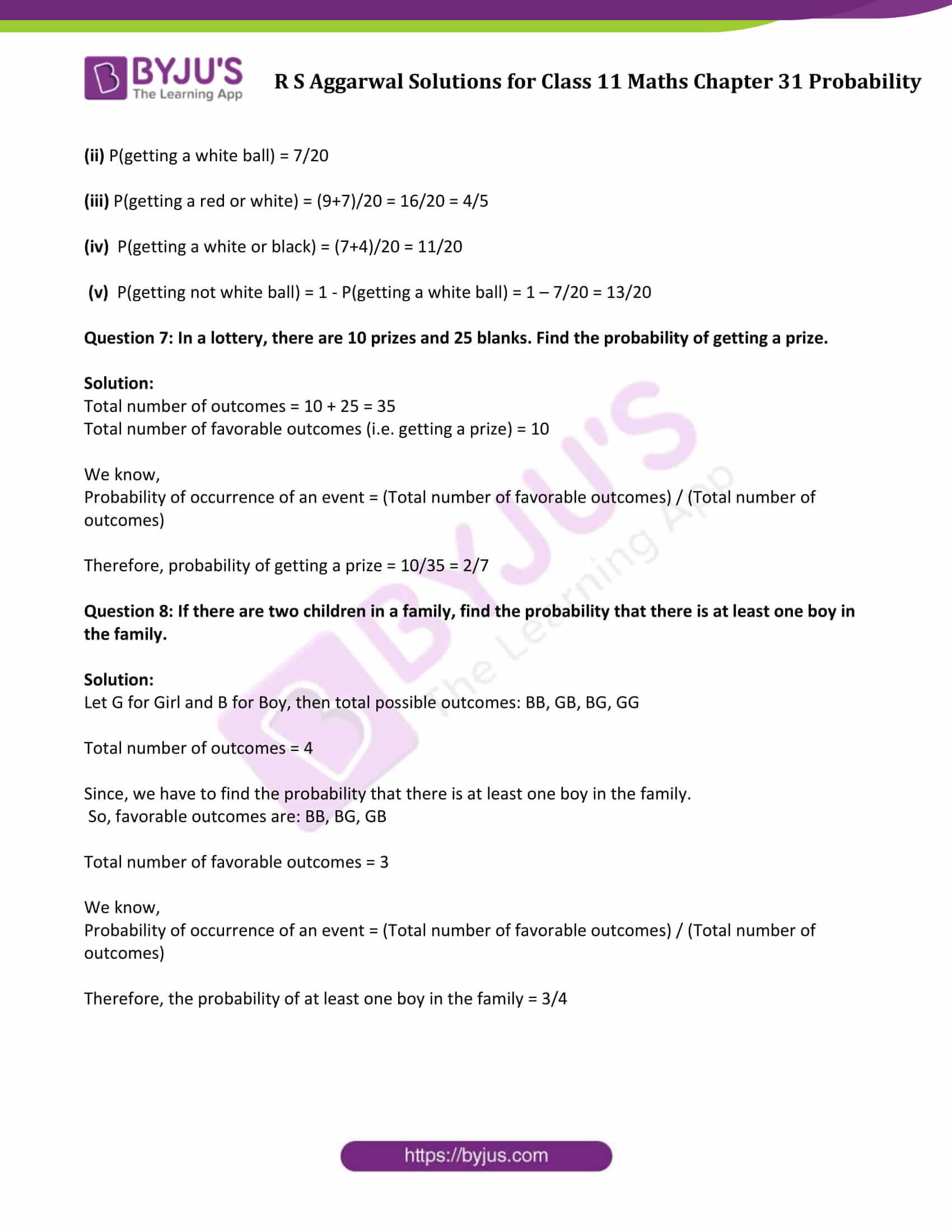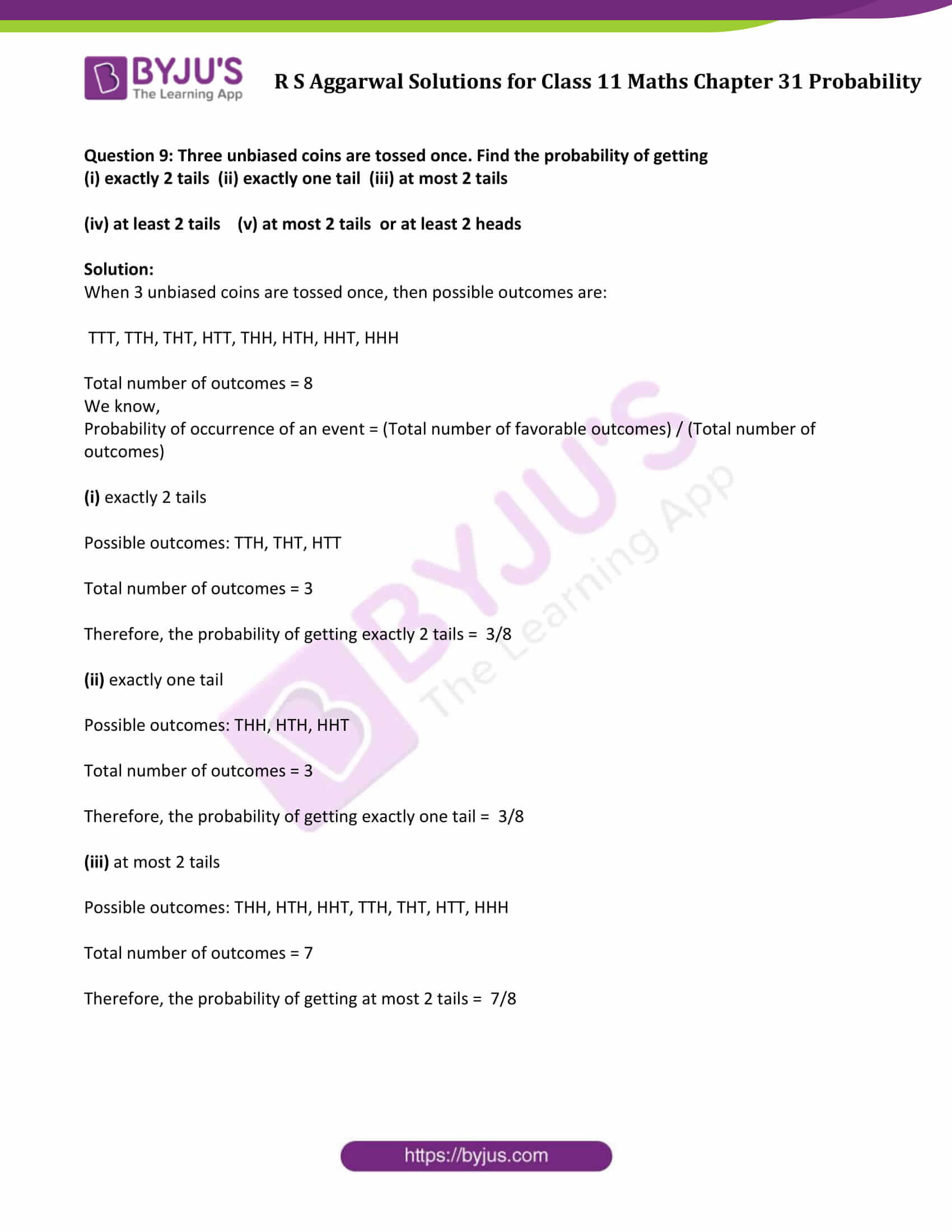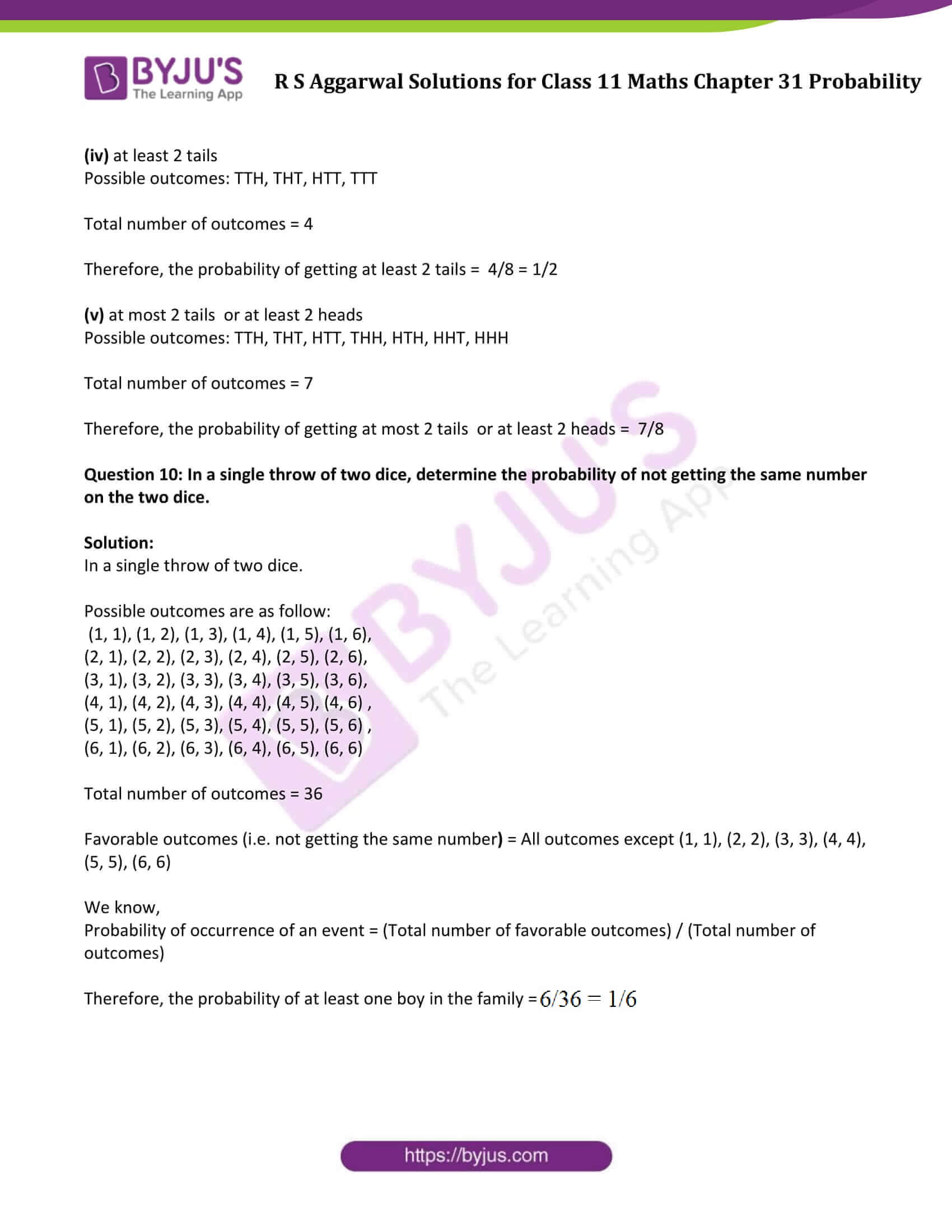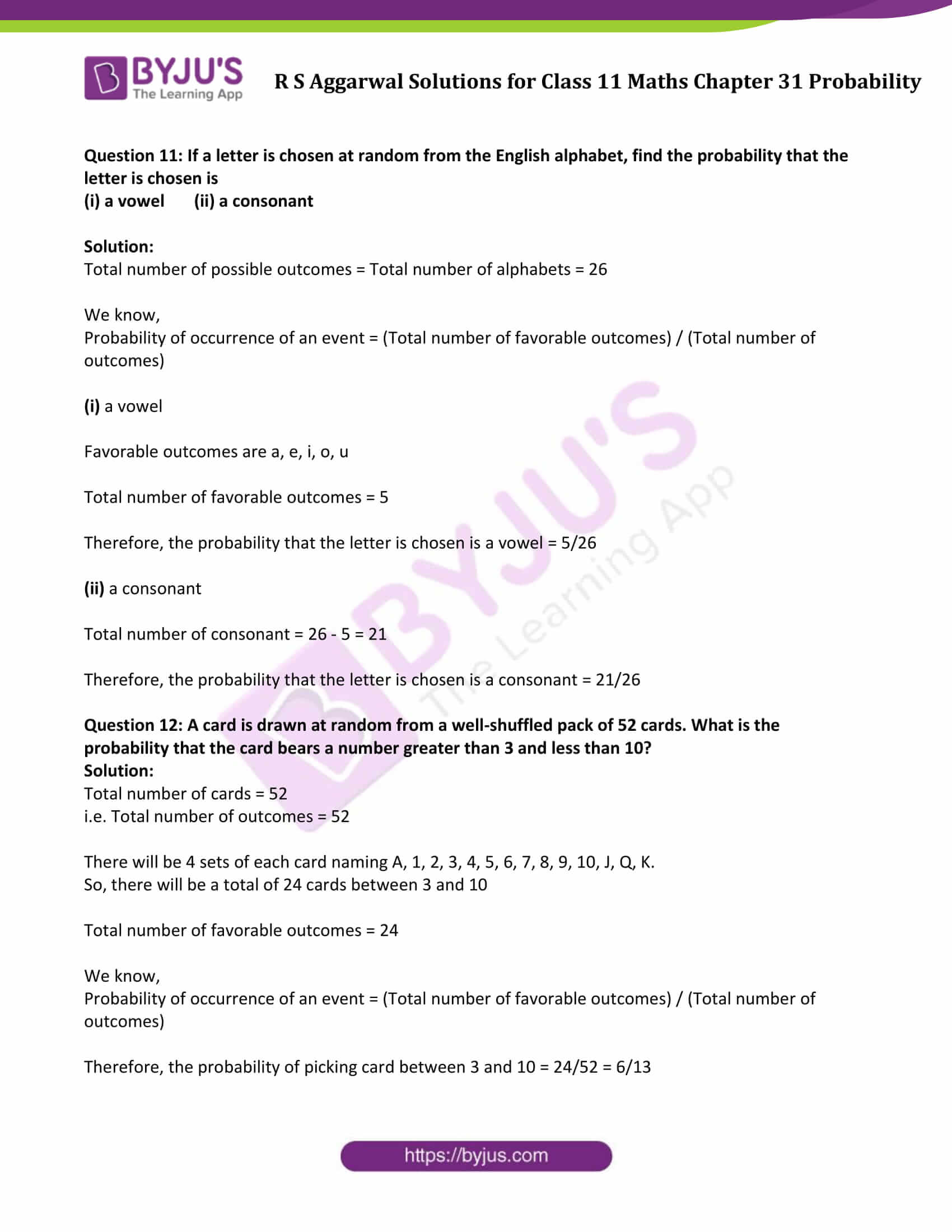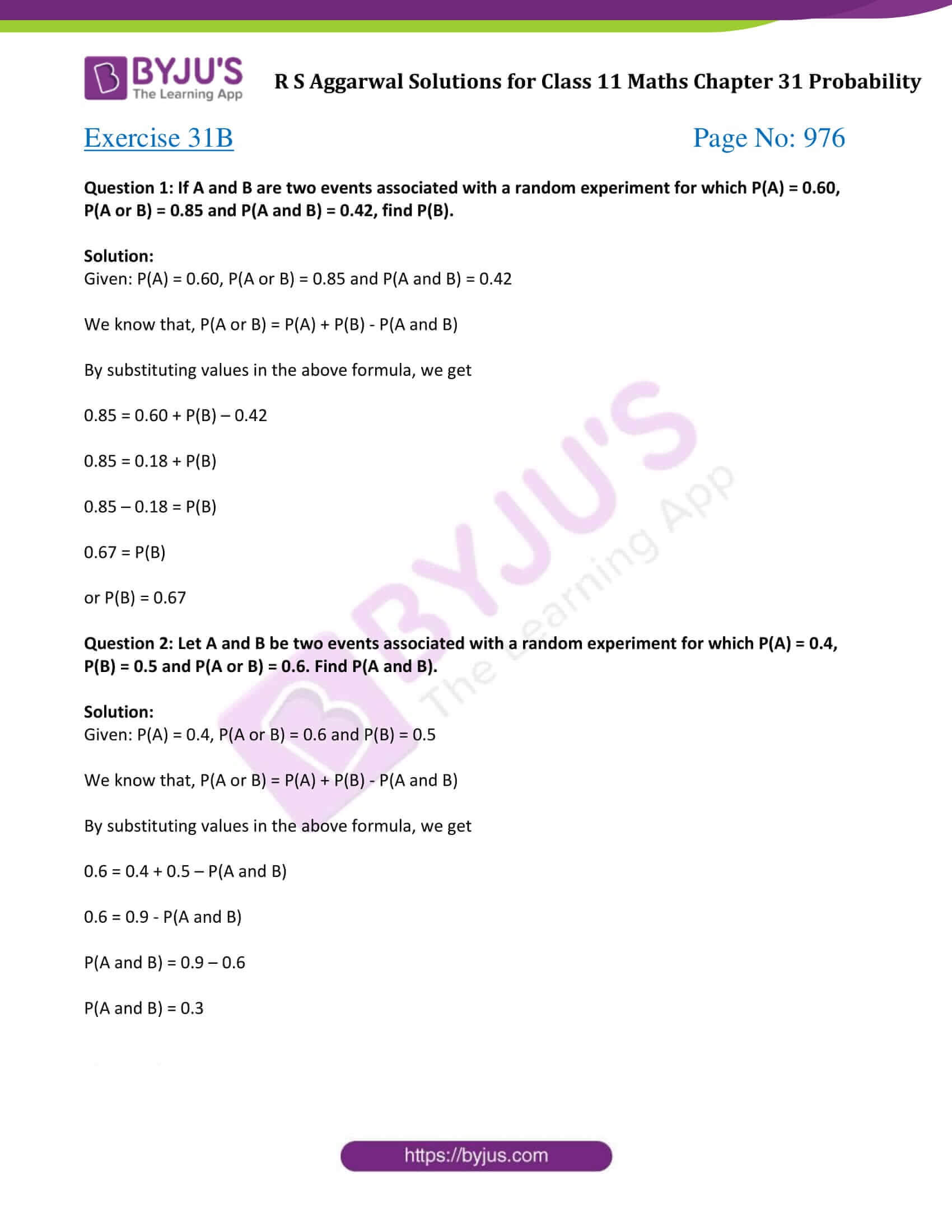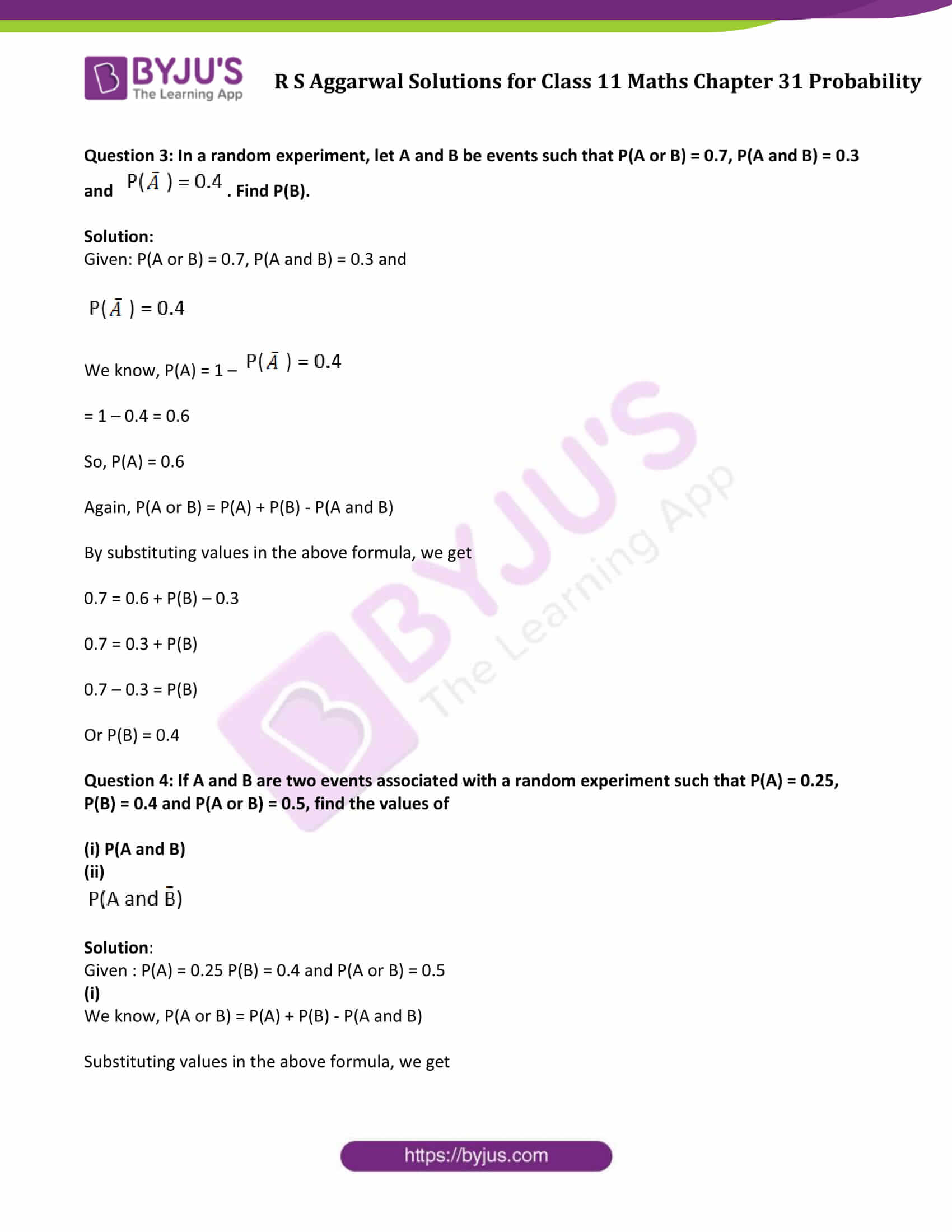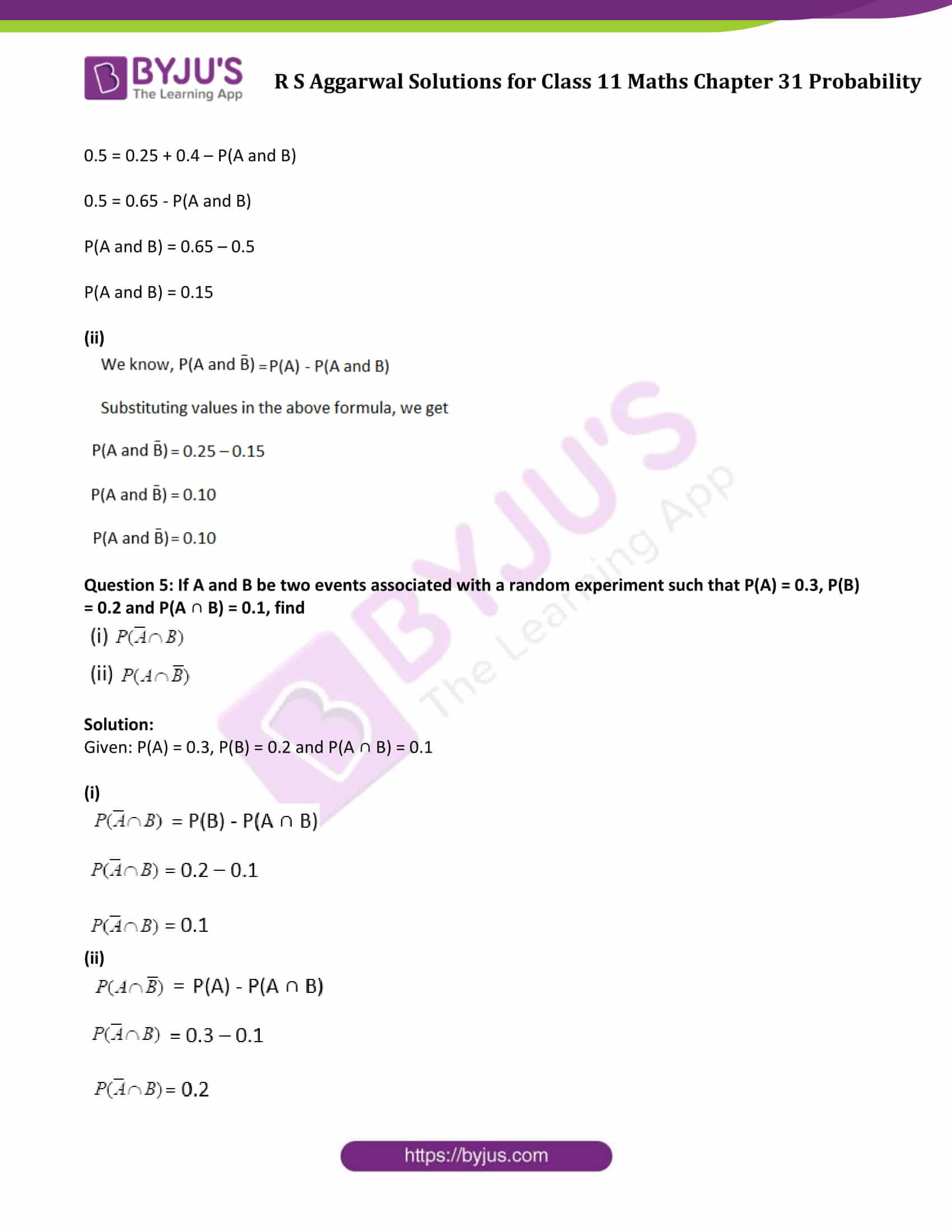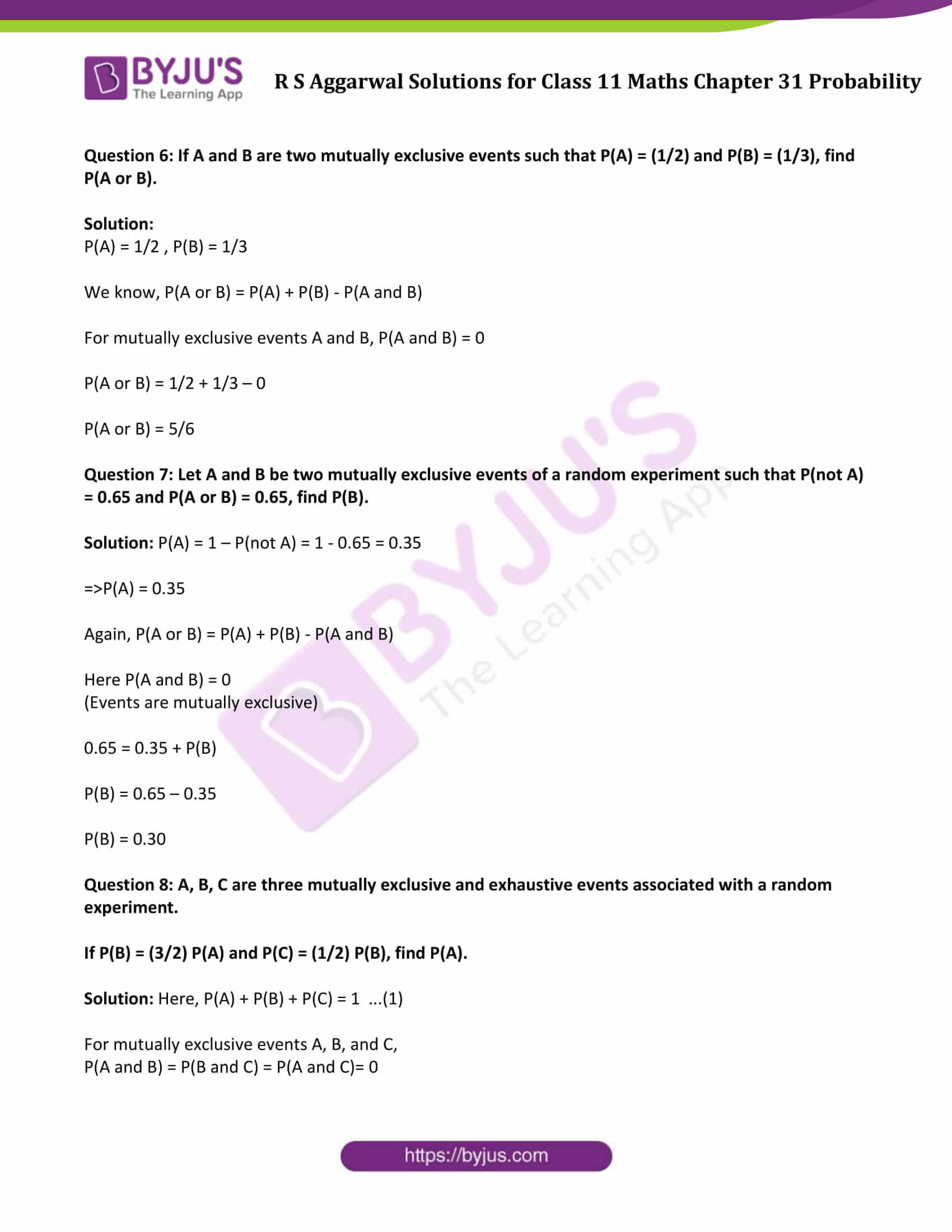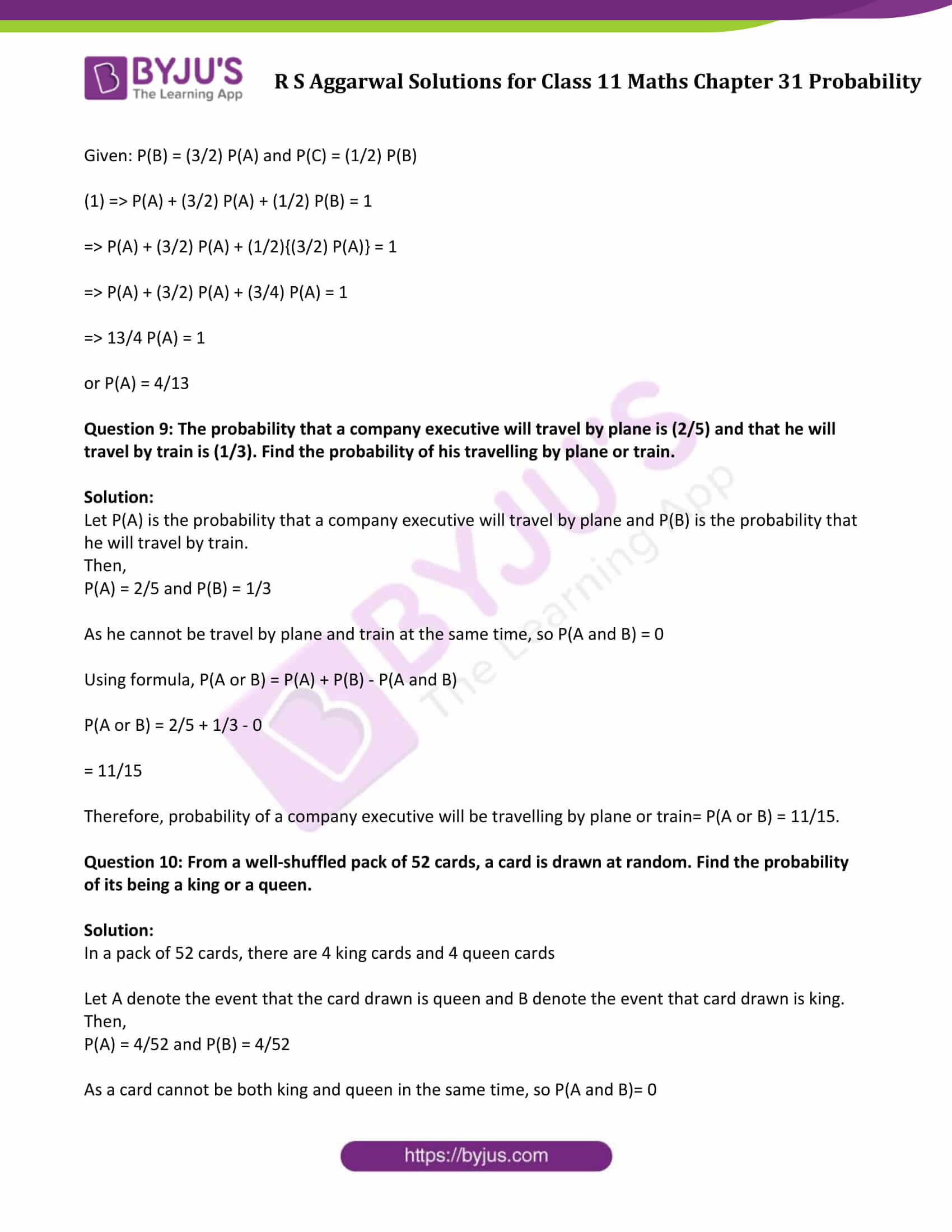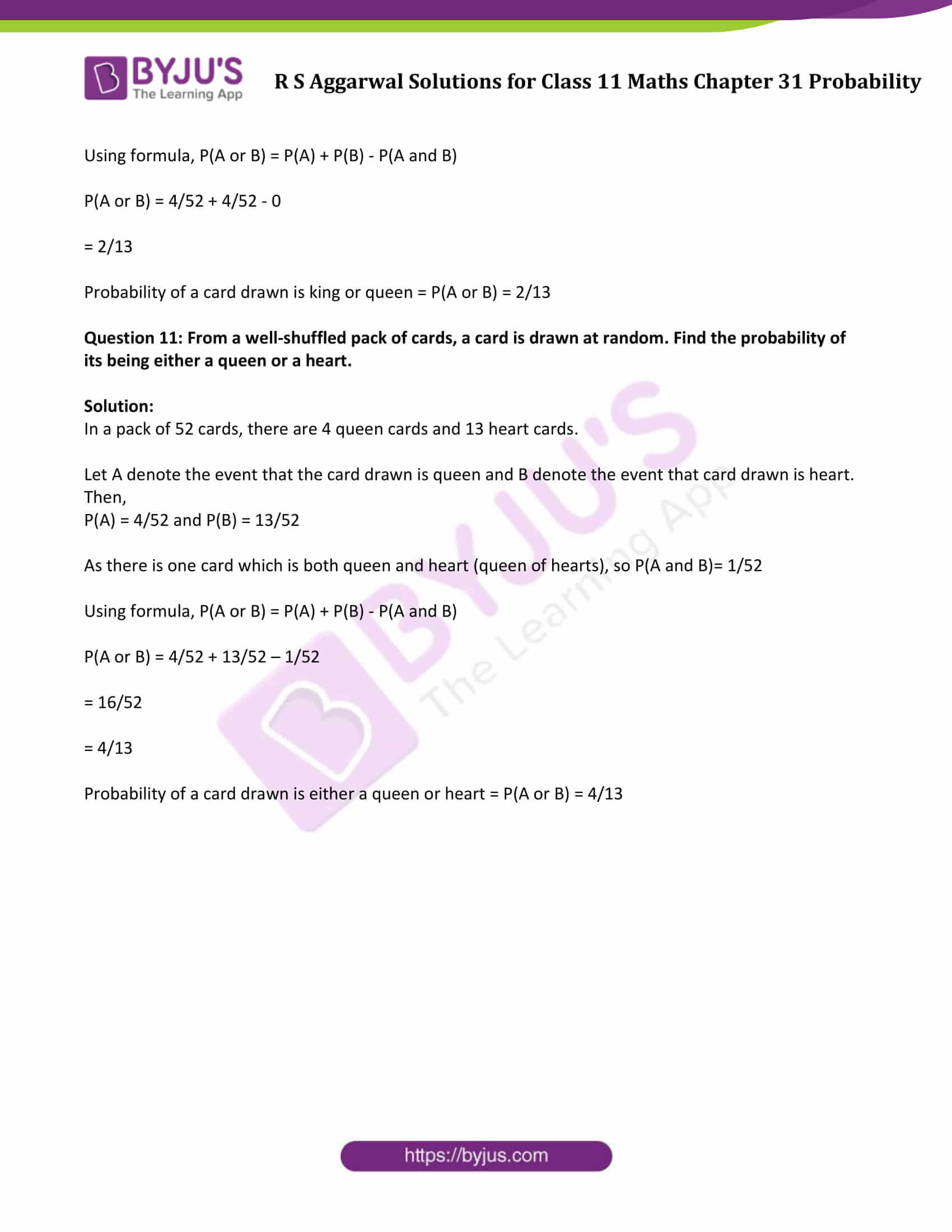### Access Answers to Maths R S Aggarwal Chapter 31 Probability

Exercise 31A Page No: 963

Question 1: A coin is tossed once. Find the probability of getting a tail.

Solution:

We know,

Probability of occurrence of an event = (Total number of favorable outcomes) / (Total number of outcomes)

In this case:

Total number of outcomes = {H, T} = 2

Number of outcomes in which tail comes = 1

Now, Probability (getting a tail) = 1/2

Question 2: A die is thrown. Find the probability of

(i) getting a 5

(ii) getting a 2 or a 3

(iii) getting an odd number

(iv) getting a prime number

(v) getting a multiple of 3

(vi) getting a number between 3 and 6

Solution:

We know,

Probability of occurrence of an event = (Total number of favorable outcomes) / (Total number of outcomes)

Total outcomes are 1, 2, 3, 4, 5, 6.

Total number of outcomes = 6

(i) getting a 5

Total number of desired outcomes i.e. getting 5 = 1

Probability (getting a 5) = 1/6

(ii) getting a 2 or a 3

Total number of desired outcomes i.e. getting 2 or 3 = 2

Probability (getting 2 or 3) = 2/6 = 1/3

(iii) getting an odd number

Total number of desired outcomes i.e. an odd number = 1, 3, 5 = 3

Probability (getting an odd number) = 3/6 = 1/2

(iv) getting a prime number

Total number of desired outcomes i.e. prime number = 2, 3, 5 = 3

Probability (getting a prime number) = 3/6 = 1/2

(v) getting a multiple of 3

Multiple of 3 = 3, 6

Total number of desired outcomes = 2

Probability (getting a multiple of 3) = 2/6 = 1/3

(vi) getting a number between 3 and 6

Number between 3 and 6 = 4, 5

Total number of desired outcomes = 2

Probability (getting a number between 3 and 6) = 2/6 = 1/3

Question 3: In a single throw of two dice, find the probability of

(i) getting a sum less than 6

(ii) getting a doublet of odd numbers

(iii) getting the sum as a prime number

Solution:

Probability of occurrence of an event = (Total number of favorable outcomes) / (Total number of outcomes)

Possible outcomes are as follow:

(1, 1), (1, 2), (1, 3), (1, 4), (1, 5), (1, 6),

(2, 1), (2, 2), (2, 3), (2, 4), (2, 5), (2, 6),

(3, 1), (3, 2), (3, 3), (3, 4), (3, 5), (3, 6),

(4, 1), (4, 2), (4, 3), (4, 4), (4, 5), (4, 6) ,

(5, 1), (5, 2), (5, 3), (5, 4), (5, 5), (5, 6) ,

(6, 1), (6, 2), (6, 3), (6, 4), (6, 5), (6, 6)

Total number of outcomes = 36

(i) getting a sum less than 6

Pick entries having sum less than 6:

(1, 1), (1, 2), (1, 3), (1, 4), (2, 1), (2, 2), (2, 3), (3, 1), (3, 2), (4, 1)

Total number of favorable outcomes = 10

Probability (getting a sum less than 6) = 10/36 or 5/18

(ii) getting a doublet of odd numbers

Pick entries having doublet of odd numbers:

(1, 1), (3, 3), (5, 5)

Total number of favorable outcomes = 3

Probability (getting a doublet of odd numbers) = 3/36 or 1/12

(iii) getting the sum as a prime number

Pick entries having sum as a prime number:

(1, 1), (1, 2), (1, 4), (1, 6), (2, 1), (2, 3), (2, 5), (3, 2), (3, 4), (4, 1), (4, 3), (5, 2), (5, 6), (6, 1), (6, 5)

Total number of favorable outcomes = 15

Probability (getting the sum as a prime number) = 15/36 or 5/12

Question 4: In a single throw of two dice, find

(i) P (an odd number on the first die and a 6 on the second)

(ii) P (a number greater than 3 on each die)

(iii) P (a total of 10)

(iv) P (a total greater than 8)

(v) P (a total of 9 or 11)

Solution:

Probability of occurrence of an event = (Total number of favorable outcomes) / (Total number of outcomes)

Possible outcomes are as follow:

(1, 1), (1, 2), (1, 3), (1, 4), (1, 5), (1, 6),

(2, 1), (2, 2), (2, 3), (2, 4), (2, 5), (2, 6),

(3, 1), (3, 2), (3, 3), (3, 4), (3, 5), (3, 6),

(4, 1), (4, 2), (4, 3), (4, 4), (4, 5), (4, 6),

(5, 1), (5, 2), (5, 3), (5, 4), (5, 5), (5, 6),

(6, 1), (6, 2), (6, 3), (6, 4), (6, 5), (6, 6)

Total number of outcomes = 36

(i) Pick favorable entries: (1, 6), (3, 6), (5, 6)

Total number of favorable outcomes = 3

P (an odd number on the first die and a 6 on the second) = 3/36 = 1/12

(ii) Pick favorable entries: (4, 4), (4, 5), (4, 6), (5, 4), (5, 5), (5, 6), (6, 4), (6, 5), (6, 6)

Total number of favorable outcomes = 9

P (a number greater than 3 on each die) = 9/36 = 1/4

(iii) Pick favorable entries: (4, 6), (5, 5), (6, 4)

Total number of favorable outcomes = 3

P (a total of 10) = 3/36 = 1/12

(iv) Pick favorable entries: (3, 6), (4, 5), (4, 6), (5, 4), (5, 5), (5, 6), (6, 3), (6, 4), (6, 5), (6, 6)

Total number of favorable outcomes = 10

P (a total greater than 8) = 10/36 = 5/18

(v) Pick favorable entries: (3, 6), (4, 5), (5, 4), (6, 3), (6, 5) , (5, 6)

Total number of favorable outcomes = 6

P (a total of 9 or 11) = 6/36 = 1/6

Question 5: A bag contains 4 white and 5 black balls. A ball is drawn at random from the bag. Find the probability that the ball is drawn is white.

Solution:

Given: A bag contains 4 white and 5 black balls

Total number of balls = 4 + 5 = 9

Total number of white balls = 4

We know,

Probability of occurrence of an event = (Total number of favorable outcomes) / (Total number of outcomes)

So, P(getting white ball) = 4/9

Question 6: An urn contains 9 red, 7 white, and 4 black balls. A ball is drawn at random. Find the probability that the ball is drawn is

(i) red (ii) white (iii) red or white (iv) white or black (v) not white

Solution:

Total number of Red balls = 9

Total number of white balls = 7

Total number of black balls = 4

Total number of balls = 9 + 7 + 4 = 20

We know,

Probability of occurrence of an event = (Total number of favorable outcomes) / (Total number of outcomes)

(i) P(getting a red ball) = 9/20

(ii) P(getting a white ball) = 7/20

(iii) P(getting a red or white) = (9+7)/20 = 16/20 = 4/5

(iv) P(getting a white or black) = (7+4)/20 = 11/20

(v) P(getting not white ball) = 1 – P(getting a white ball) = 1 – 7/20 = 13/20

Question 7: In a lottery, there are 10 prizes and 25 blanks. Find the probability of getting a prize.

Solution:

Total number of outcomes = 10 + 25 = 35

Total number of favorable outcomes (i.e. getting a prize) = 10

We know,

Probability of occurrence of an event = (Total number of favorable outcomes) / (Total number of outcomes)

Therefore, probability of getting a prize = 10/35 = 2/7

Question 8: If there are two children in a family, find the probability that there is at least one boy in the family.

Solution:

Let G for Girl and B for Boy, then total possible outcomes: BB, GB, BG, GG

Total number of outcomes = 4

Since, we have to find the probability that there is at least one boy in the family.

So, favorable outcomes are: BB, BG, GB

Total number of favorable outcomes = 3

We know,

Probability of occurrence of an event = (Total number of favorable outcomes) / (Total number of outcomes)

Therefore, the probability of at least one boy in the family = 3/4

Question 9: Three unbiased coins are tossed once. Find the probability of getting

(i) exactly 2 tails (ii) exactly one tail (iii) at most 2 tails

(iv) at least 2 tails (v) at most 2 tails or at least 2 heads

Solution:

When 3 unbiased coins are tossed once, then possible outcomes are:

TTT, TTH, THT, HTT, THH, HTH, HHT, HHH

Total number of outcomes = 8

We know,

Probability of occurrence of an event = (Total number of favorable outcomes) / (Total number of outcomes)

(i) exactly 2 tails

Possible outcomes: TTH, THT, HTT

Total number of outcomes = 3

Therefore, the probability of getting exactly 2 tails = 3/8

(ii) exactly one tail

Possible outcomes: THH, HTH, HHT

Total number of outcomes = 3

Therefore, the probability of getting exactly one tail = 3/8

(iii) at most 2 tails

Possible outcomes: THH, HTH, HHT, TTH, THT, HTT, HHH

Total number of outcomes = 7

Therefore, the probability of getting at most 2 tails = 7/8

(iv) at least 2 tails

Possible outcomes: TTH, THT, HTT, TTT

Total number of outcomes = 4

Therefore, the probability of getting at least 2 tails = 4/8 = 1/2

(v) at most 2 tails or at least 2 heads

Possible outcomes: TTH, THT, HTT, THH, HTH, HHT, HHH

Total number of outcomes = 7

Therefore, the probability of getting at most 2 tails or at least 2 heads = 7/8

Question 10: In a single throw of two dice, determine the probability of not getting the same number on the two dice.

Solution:

In a single throw of two dice.

Possible outcomes are as follow:

(1, 1), (1, 2), (1, 3), (1, 4), (1, 5), (1, 6),

(2, 1), (2, 2), (2, 3), (2, 4), (2, 5), (2, 6),

(3, 1), (3, 2), (3, 3), (3, 4), (3, 5), (3, 6),

(4, 1), (4, 2), (4, 3), (4, 4), (4, 5), (4, 6) ,

(5, 1), (5, 2), (5, 3), (5, 4), (5, 5), (5, 6) ,

(6, 1), (6, 2), (6, 3), (6, 4), (6, 5), (6, 6)

Total number of outcomes = 36

Favorable outcomes (i.e. not getting the same number) = All outcomes except (1, 1), (2, 2), (3, 3), (4, 4), (5, 5), (6, 6)

We know,

Probability of occurrence of an event = (Total number of favorable outcomes) / (Total number of outcomes)

Therefore, the probability of at least one boy in the family = 6/36 = 1/6

Question 11: If a letter is chosen at random from the English alphabet, find the probability that the letter is chosen is

(i) a vowel (ii) a consonant

Solution:

Total number of possible outcomes = Total number of alphabets = 26

We know,

Probability of occurrence of an event = (Total number of favorable outcomes) / (Total number of outcomes)

(i) a vowel

Favorable outcomes are a, e, i, o, u

Total number of favorable outcomes = 5

Therefore, the probability that the letter is chosen is a vowel = 5/26

(ii) a consonant

Total number of consonant = 26 – 5 = 21

Therefore, the probability that the letter is chosen is a consonant = 21/26

Question 12: A card is drawn at random from a well-shuffled pack of 52 cards. What is the probability that the card bears a number greater than 3 and less than 10?

Solution:

Total number of cards = 52

i.e. Total number of outcomes = 52

There will be 4 sets of each card naming A, 1, 2, 3, 4, 5, 6, 7, 8, 9, 10, J, Q, K.

So, there will be a total of 24 cards between 3 and 10

Total number of favorable outcomes = 24

We know,

Probability of occurrence of an event = (Total number of favorable outcomes) / (Total number of outcomes)

Therefore, the probability of picking card between 3 and 10 = 24/52 = 6/13

Exercise 31B Page No: 976

Question 1: If A and B are two events associated with a random experiment for which P(A) = 0.60, P(A or B) = 0.85 and P(A and B) = 0.42, find P(B).

Solution:

Given: P(A) = 0.60, P(A or B) = 0.85 and P(A and B) = 0.42

We know that, P(A or B) = P(A) + P(B) – P(A and B)

By substituting values in the above formula, we get

0.85 = 0.60 + P(B) – 0.42

0.85 = 0.18 + P(B)

0.85 – 0.18 = P(B)

0.67 = P(B)

or P(B) = 0.67

Question 2: Let A and B be two events associated with a random experiment for which P(A) = 0.4, P(B) = 0.5 and P(A or B) = 0.6. Find P(A and B).

Solution:

Given: P(A) = 0.4, P(A or B) = 0.6 and P(B) = 0.5

We know that, P(A or B) = P(A) + P(B) – P(A and B)

By substituting values in the above formula, we get

0.6 = 0.4 + 0.5 – P(A and B)

0.6 = 0.9 – P(A and B)

P(A and B) = 0.9 – 0.6

P(A and B) = 0.3

Question 3: In a random experiment, let A and B be events such that P(A or B) = 0.7, P(A and B) = 0.3 and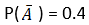. Find P(B).

Solution:

Given: P(A or B) = 0.7, P(A and B) = 0.3 andWe know, P(A) = 1 –= 1 – 0.4 = 0.6

So, P(A) = 0.6

Again, P(A or B) = P(A) + P(B) – P(A and B)

By substituting values in the above formula, we get

0.7 = 0.6 + P(B) – 0.3

0.7 = 0.3 + P(B)

0.7 – 0.3 = P(B)

Or P(B) = 0.4

Question 4: If A and B are two events associated with a random experiment such that P(A) = 0.25, P(B) = 0.4 and P(A or B) = 0.5, find the values of

(i) P(A and B)

(ii)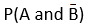Solution:

Given : P(A) = 0.25 P(B) = 0.4 and P(A or B) = 0.5

(i)

We know, P(A or B) = P(A) + P(B) – P(A and B)

Substituting values in the above formula, we get

0.5 = 0.25 + 0.4 – P(A and B)

0.5 = 0.65 – P(A and B)

P(A and B) = 0.65 – 0.5

P(A and B) = 0.15

(ii)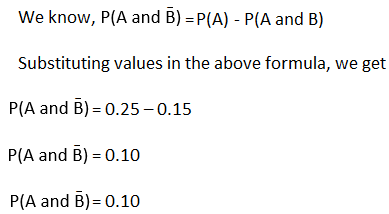Question 5: If A and B be two events associated with a random experiment such that P(A) = 0.3, P(B) = 0.2 and P(A ∩ B) = 0.1, find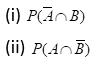Solution:

Given: P(A) = 0.3, P(B) = 0.2 and P(A ∩ B) = 0.1

(i)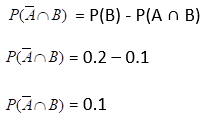(ii)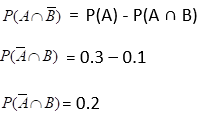Question 6: If A and B are two mutually exclusive events such that P(A) = (1/2) and P(B) = (1/3), find P(A or B).

Solution:

P(A) = 1/2 , P(B) = 1/3

We know, P(A or B) = P(A) + P(B) – P(A and B)

For mutually exclusive events A and B, P(A and B) = 0

P(A or B) = 1/2 + 1/3 – 0

P(A or B) = 5/6

Question 7: Let A and B be two mutually exclusive events of a random experiment such that P(not A) = 0.65 and P(A or B) = 0.65, find P(B).

Solution: P(A) = 1 – P(not A) = 1 – 0.65 = 0.35

=>P(A) = 0.35

Again, P(A or B) = P(A) + P(B) – P(A and B)

Here P(A and B) = 0

(Events are mutually exclusive)

0.65 = 0.35 + P(B)

P(B) = 0.65 – 0.35

P(B) = 0.30

Question 8: A, B, C are three mutually exclusive and exhaustive events associated with a random experiment.

If P(B) = (3/2) P(A) and P(C) = (1/2) P(B), find P(A).

Solution: Here, P(A) + P(B) + P(C) = 1 …(1)

For mutually exclusive events A, B, and C,

P(A and B) = P(B and C) = P(A and C)= 0

Given: P(B) = (3/2) P(A) and P(C) = (1/2) P(B)

(1) => P(A) + (3/2) P(A) + (1/2) P(B) = 1

=> P(A) + (3/2) P(A) + (1/2){(3/2) P(A)} = 1

=> P(A) + (3/2) P(A) + (3/4) P(A) = 1

=> 13/4 P(A) = 1

or P(A) = 4/13

Question 9: The probability that a company executive will travel by plane is (2/5) and that he will travel by train is (1/3). Find the probability of his travelling by plane or train.

Solution:

Let P(A) is the probability that a company executive will travel by plane and P(B) is the probability that he will travel by train.

Then,

P(A) = 2/5 and P(B) = 1/3

As he cannot be travel by plane and train at the same time, so P(A and B) = 0

Using formula, P(A or B) = P(A) + P(B) – P(A and B)

P(A or B) = 2/5 + 1/3 – 0

= 11/15

Therefore, probability of a company executive will be travelling by plane or train= P(A or B) = 11/15.

Question 10: From a well-shuffled pack of 52 cards, a card is drawn at random. Find the probability of its being a king or a queen.

Solution:

In a pack of 52 cards, there are 4 king cards and 4 queen cards

Let A denote the event that the card drawn is queen and B denote the event that card drawn is king.

Then,

P(A) = 4/52 and P(B) = 4/52

As a card cannot be both king and queen in the same time, so P(A and B)= 0

Using formula, P(A or B) = P(A) + P(B) – P(A and B)

P(A or B) = 4/52 + 4/52 – 0

= 2/13

Probability of a card drawn is king or queen = P(A or B) = 2/13

Question 11: From a well-shuffled pack of cards, a card is drawn at random. Find the probability of its being either a queen or a heart.

Solution:

In a pack of 52 cards, there are 4 queen cards and 13 heart cards.

Let A denote the event that the card drawn is queen and B denote the event that card drawn is heart.

Then,

P(A) = 4/52 and P(B) = 13/52

As there is one card which is both queen and heart (queen of hearts), so P(A and B)= 1/52

Using formula, P(A or B) = P(A) + P(B) – P(A and B)

P(A or B) = 4/52 + 13/52 – 1/52

= 16/52

= 4/13

Probability of a card drawn is either a queen or heart = P(A or B) = 4/13

## R S Aggarwal Solutions For Class 11 Maths Chapter 31 Exercises:

Get detailed solutions for all the questions listed under below exercises:

Exercise 31A Solutions

Exercise 31B Solutions